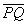Mathematics

# isosceles Triangle

In Geometry, An isosceles triangle is a polygon having three sides. it has a pair of equal and remaining one of the unequallength sides. according to the lengths of sides, the isosceles triangle considers a special case from 6 different types of triangles. for example, in the following figure, line a is equal to the opposite line a. Hence, angles formed by these two sides will also be equal to each other and is known as vertex angle.

• angles of isosceles Triangle
• isosceles Triangle formula
• area of isosceles Triangle
• different properties
• theorem

So, if you want the complete information, start this 5-minute journey with us.

Let’s Get Started…!

## Overview

the word isosceles is derived from Greek terms “isos” (equal) and “Skelos” (leg). it is a polygon which is a two-dimensional geometric figure made with the union of different points (not on the same line) these points are joined by line segments.

hence, the isosceles Triangle is said to be a closed shape or space. the word “isosceles” can also be used for other geometric figures like a trapezoid. in this case, a trapezoid having two equal sides is known as an isosceles trapezoid.

## What are the 4 basic elements of the isosceles triangle?

they are as follows:

1. Vertices: from the above figure, a,b and c are known as vertices.
2. Sides: A, B, and C are the sides of the triangle.
3. Interior Angles: α, β and γ are the interior angles that always give the sum of 180º by adding them up.
4. Exterior Angles: x, y, z are said to be exterior angles or supplementary angles to the angles of the same interior side. in other words, adding up any two angles will result in 180º.

## Types of Isosceles triangles

there are three basic types:

• Acute angle: in which all angles are less than 90º or acute.
• Rectangle: in which one angle is is equal to 90º while the other two are equals to 45º.
• Blunt: in which one angle is obtuse (greater than 90º) while others are acute (less than 90º).

## Isosceles triangle area

the base (b) and corresponding height (h) of the triangle helps to find the area of the Isosceles triangle.## The perimeter of an isosceles triangle

the perimeter can be calculated by adding up three sides of the triangle. we use Heron’s formula to calculate a semi perimeter, s = P / 2. where s is the perimeter. suppose that two sides of a triangle are equal in length, then, we double the perimeter of side (a) and add with uneven side ( b ).if the value of these repeating sides (say a) and angle formed between them is known, we can find the other unknown side (say b) by using the cosine theorem.## Properties

• if the two sides are equal in length, then their consequent angle will also be equal.
• if the base of the triangle is different, the vertex will also be different from the other adjacent side’s angle.
• the triangle that has two bisectors also called an isosceles triangle and the bisector of its base is the axis of symmetry.
• both equal opposite sides always have less than 90º or acute angles.

## Isosceles triangle theorem

this theorem states that if If two sides of a triangle are congruent, then the angles opposite those sides are congruent.Proof:

Let’s say that S is the midpoint ofand R and S.Since S is the midpoint of PQ,

For the Reflective Property,

It is given that

Therefore, by LLL, Since the corresponding parts of congruent triangles are congruent.

What are the rules of an isosceles triangle?

Ans: followings are the properties and rules for the isosceles:

• two sides will be congruent to each other.
• the third side which is unequal to congruent will call the base of the triangle.
• the angles between the congruent sides will also be equal to each other.

Do isosceles triangles add up to 180?

Ans: A triangle always consists of three sides. two equal lengths sides are opposite to each other are called the legs of the triangle while the third side is known as the base. the angle between equal sides is said to be vertex angle. it is necessary that when adding up all three sides should have resulted as 180º.

What is the formula of isosceles?

Ans: the formula of isosceles is called the area of a triangle which can be found by using its three sides. we suppose that two adjacent equal sides are named as a and b respectively, while the third side c is base. so,

Area = 2.a +b

Which angles are equal in an isosceles triangle?

An: the two equal sides and two equal angles are always equal to each other respectively.

What are the 4 types of triangles?

Ans: we have 6 different kinds of triangles as equilateral triangles, right triangles, scalene triangles, obtuse triangles, acute triangles, and isosceles triangles.

What two features make an isosceles triangle?

Ans: two equal sides and two angles equal to each other respectively make an isosceles triangle.

How do you prove a triangle is an isosceles triangle?

Ans: if two sides are equal to each other and make an acute angle, then the triangle will be an isosceles triangle.

You May Also Like: